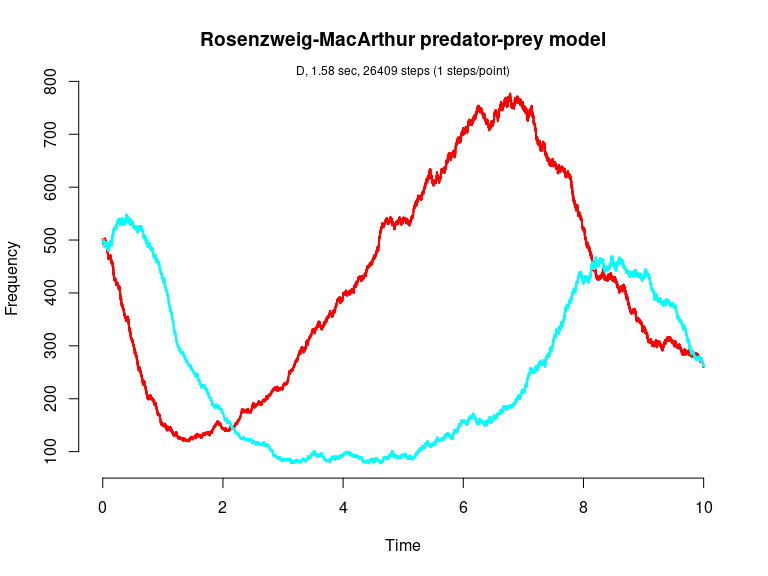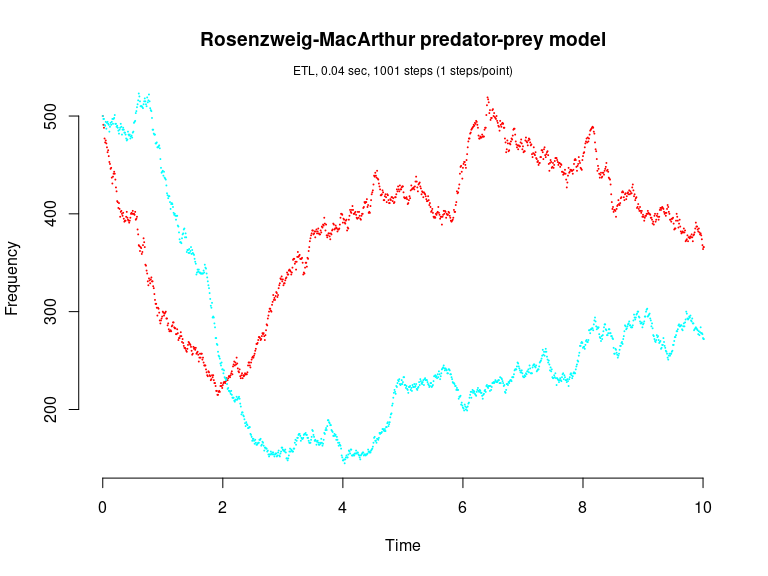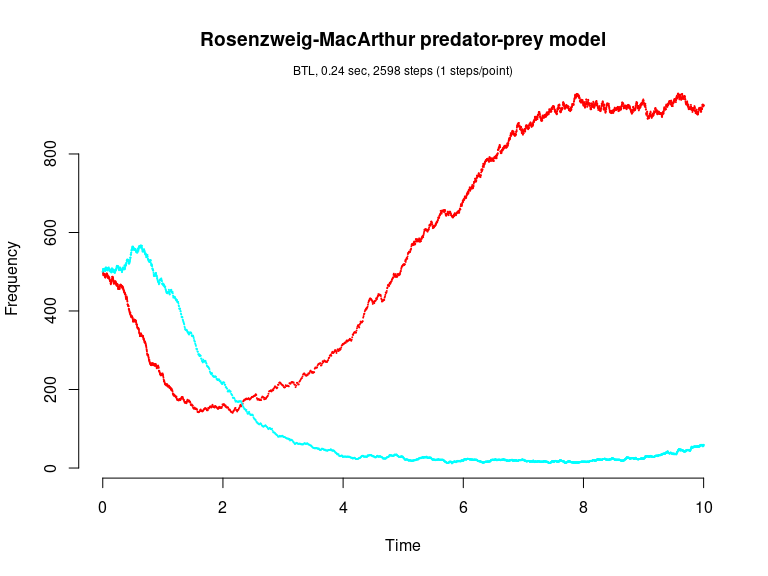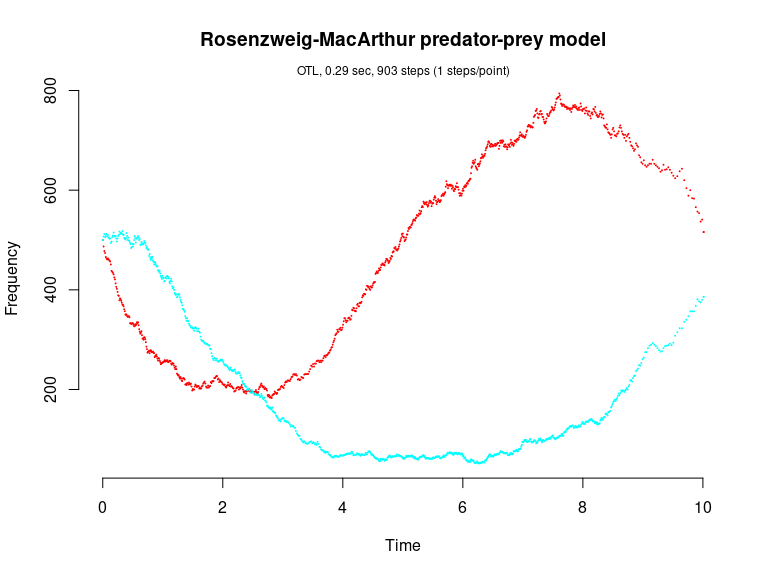# Rosenzweig-MacArthur predator-prey model (Pineda-Krch et al., 2007)

Rosenzweig-MacArthur predator-prey model (Pineda-Krch et al., 2007, Pineda-Krch, 2008)

dN/dt = r(1-N/K - alpha/(1+wN))NP
dP/dt = c*alpha/(1+wN))NP

This model has five reactions with the following per capita rates,

prey birth:     b
prey death:     d+(b-d)N/K
predation:      alpha/(1+wN)
predator birth: c*alpha/(1+wN)N
predator death: g

Propensity functions:

a1 = b * N
a2 = (d+(b-d)N/K) * N
a3 = alpha/(1+wN) * N * P
a4 = c*alpha/(1+wN) * N * P
a5 = g * P

library(GillespieSSA)

Define parameters

parms <- c(b=2, d=1, K=1000, alpha=0.005,
w=0.0025, c=2, g=2)
tf <- 10                                               # Final time
simName <- "Rosenzweig-MacArthur predator-prey model"  # Name

Define initial state vector

x0  <- c(N=500, P=500)

Define state-change matrix

nu  <- matrix(c(+1, -1, -1,  0,  0,
0,  0,  0, +1, -1),
nrow=2,byrow=TRUE) 

Define propensity functions

a <- c(
"b*N",
"(d+(b-d)*N/K)*N",
"alpha/(1+w*N)*N*P",
"c*alpha/(1+w*N)*N*P",
"g*P"
) 

Run simulations with the Direct method

set.seed(1)
out <- ssa(
x0 = x0,
a = a,
nu = nu,
parms = parms,
tf = tf,
method = ssa.d(),
simName = simName,
verbose = FALSE,
consoleInterval = 1
)
ssa.plot(out, show.title = TRUE, show.legend = FALSE)Run simulations with the Explict tau-leap method

set.seed(1)
out <- ssa(
x0 = x0,
a = a,
nu = nu,
parms = parms,
tf = tf,
method = ssa.etl(tau = .01),
simName = simName,
verbose = FALSE,
consoleInterval = 1
)
ssa.plot(out, show.title = TRUE, show.legend = FALSE)Run simulations with the Binomial tau-leap method

set.seed(1)
out <- ssa(
x0 = x0,
a = a,
nu = nu,
parms = parms,
tf = tf,
method = ssa.btl(),
simName = simName,
verbose = FALSE,
consoleInterval = 1
)
ssa.plot(out, show.title = TRUE, show.legend = FALSE)Run simulations with the Optimized tau-leap method

set.seed(1)
out <- ssa(
x0 = x0,
a = a,
nu = nu,
parms = parms,
tf = tf,
method = ssa.otl(),
simName = simName,
verbose = FALSE,
consoleInterval = 1
)
ssa.plot(out, show.title = TRUE, show.legend = FALSE)